# Quantum homogeneous space

(diff) ← Older revision | Latest revision (diff) | Newer revision → (diff)

A unital algebrathat is a co-module for a quantum group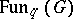(cf. Quantum groups) and for which the structure mapping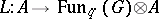is an algebra homomorphism, i.e.,is a co-module algebra [a1]. Here,is a deformation of the Poisson algebra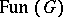, of a Poisson–Lie group, endowed with the structure of a Hopf algebra with a co-multiplicationand a co-unit. Often, bothandcan also be equipped with a-involution. The left co-actionsatisfiesThese relations should be modified correspondingly for a right co-action. In the dual picture, if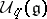is the deformed universal enveloping algebra of the Lie algebraandis a non-degenerate dual pairing between the Hopf algebrasand, then the prescription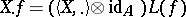, with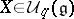and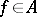, defines a right action ofon(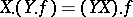) and one haswhere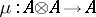is the multiplication inandis the co-multiplication in. Typically,is a deformation of the Poisson algebra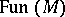(frequently called the quantization of), whereis a Poisson manifold and, at the same time, a left homogeneous space ofwith the left action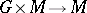a Poisson mapping.

It is not quite clear how to translate into purely algebraic terms the property thatis a homogeneous space of. One possibility is to require that only multiples of the unitsatisfy. A stronger condition requires the existence of a linear functional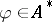such that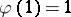while the linear mappingbe injective. Then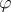can be considered as a base point.

The still stronger requirement that, in addition,be a homomorphism (a so-called classical point) holds whenis a quantization of a Poisson homogeneous spacewith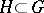a Poisson–Lie subgroup. The quantum homogeneous space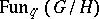is defined as the subalgebra informed by-invariant elements,where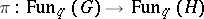is a Hopf-algebra homomorphism.

A richer class of examples is provided by quantization of orbits of the dressing transformation of, acting on its dual Poisson–Lie group (also called the generalized Pontryagin dual). The best studied cases concern the compact and solvable factorsand(andare mutually dual) in the Iwasawa decomposition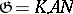, whereis a simple complex Lie group. One obtains this way, among others, the quantum sphere and, more generally, quantum Grassmannian and quantum flag manifolds.

There is a vast amount of literature on this subject. The survey book [a2] contains a rich list of references.

How to Cite This Entry:
Quantum homogeneous space. Encyclopedia of Mathematics. URL: http://encyclopediaofmath.org/index.php?title=Quantum_homogeneous_space&oldid=11401
This article was adapted from an original article by Pavel Å tovÃ­Äek (originator), which appeared in Encyclopedia of Mathematics - ISBN 1402006098. See original article